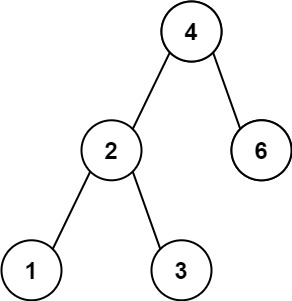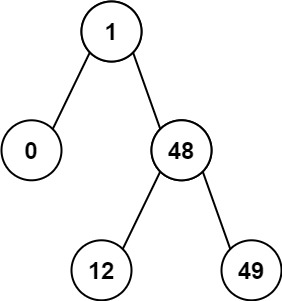# 783. Minimum Distance Between BST Nodes

Easy
Given the `root` of a Binary Search Tree (BST), return the minimum difference between the values of any two different nodes in the tree.
Example 1:Input: root = [4,2,6,1,3]
Output:
1
Example 2:Input: root = [1,0,48,null,null,12,49]
Output:
1
Constraints:
• The number of nodes in the tree is in the range `[2, 100]`.
• `0 <= Node.val <= 105`

### 解題

Runtime: 0 ms, faster than 100.00%
Memory Usage: 2.4 MB, less than 12.77%
/**
* Definition for a binary tree node.
* type TreeNode struct {
* Val int
* Left *TreeNode
* Right *TreeNode
* }
*/
func minDiffInBST(root *TreeNode) int {
arr := make([]int, 0)
res := 100000
var inorder func(*TreeNode)
inorder = func(root *TreeNode) {
if root == nil {
return
}
inorder(root.Left)
if len(arr)!=0 && root.Val - arr[len(arr)-1] < res {
res = root.Val - arr[len(arr)-1]
}
arr = append(arr, root.Val)
inorder(root.Right)
}
inorder(root)
return res
}